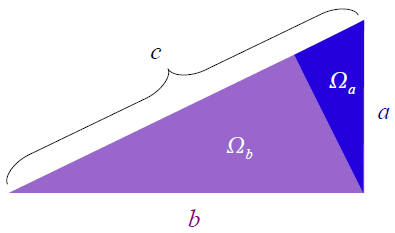## Pythagorean Theorem

The Pythagorean Theorem is one of the most important discoveries in mathematics. Here is a simple derivation (or “proof”) of the theorem. We start with a large right triangle, labeled c. Draw a perpendicular line from the right angle vertex to the hypotenuse. This subdivides the triangle c into two smaller triangles, a and b.The three triangles are similar.  This means that the areas of the triangles $$\Omega_a , \Omega_b , \Omega_c$$ obey the relation $$\displaystyle \frac{a^2}{\Omega_a} = \frac{b^2}{\Omega_b} = \frac{c^2}{\Omega_c}.$$ Furthermore, the areas have the property $$\displaystyle \Omega_c = \Omega_a + \Omega_b.$$ From the first equation we can solve for $c^2:$ $$\displaystyle c^2 = \frac{a^2}{\Omega_a} \Omega_c.$$ Using the second equation, the $\Omega_c$ can be replaced $$\displaystyle c^2 = \frac{a^2}{\Omega_a}\left(\Omega_a + \Omega_b\right)=a^2 +\frac{a^2}{\Omega_a}\Omega_b.$$ We know from the first equation that $$\displaystyle \frac{a^2}{\Omega_a} = \frac{b^2}{\Omega_b}.$$ Thus, $$\displaystyle c^2 = a^2 + b^2,$$ which is the famous result!